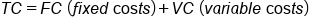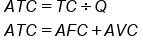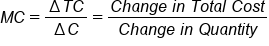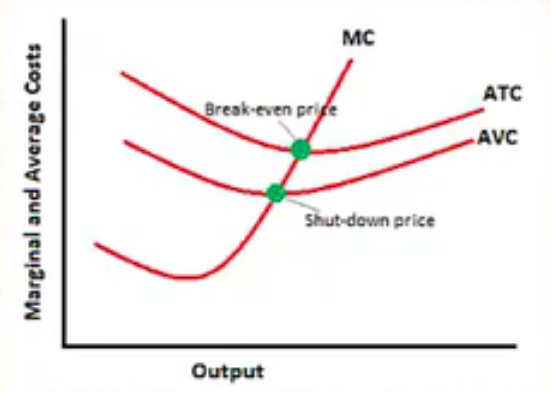+

# Cost: Total, Marginal and Average

Author: Sophia Tutorial
##### Description:

Calculate the average cost and marginal cost for a given situation.

(more)### Developing Effective Teams

*No strings attached. This college course is 100% free and is worth 1 semester credit.

37 Sophia partners guarantee credit transfer.

299 Institutions have accepted or given pre-approval for credit transfer.

* The American Council on Education's College Credit Recommendation Service (ACE Credit®) has evaluated and recommended college credit for 32 of Sophia’s online courses. Many different colleges and universities consider ACE CREDIT recommendations in determining the applicability to their course and degree programs.

Tutorial
what's covered
This tutorial will cover the different ways of looking at cost: total, marginal, and average. We will discuss the relationship between these curves and how businesses use these costs to make production decisions.

Our discussion breaks down as follows:

1. Total Cost
2. Average Cost
3. Marginal Cost
4. Relationship Between Marginal Cost and Average Cost
1. Break Even/Shut Down Points

## 1. Total Cost

As a reminder, the point of owning a business is to make a profit, and profit equals revenues minus costs.

Profit = Revenues - Costs

In this tutorial, we will focus on the cost piece of this equation.

Total cost is variable costs plus fixed costs--essentially, all of our costs.

formula
Total CostHere is a chart that reflects the various costs at different production levels.

Quantity Fixed Costs Variable Costs Total Cost
0 \$100 \$0 \$100
1 \$100 \$30 \$130
2 \$100 \$50 \$150
3 \$100 \$60 \$160
4 \$100 \$72 \$175
5 \$100 \$85 \$185
6 \$100 \$110 \$210

Notice that fixed costs are the same regardless of how much is being produced. Even when we produce nothing, we still have a fixed cost.

EXAMPLE

A good example of a fixed cost is rent. Even if you produce nothing, you still have to pay your rent.

Variable costs, on the other hand, vary with production; they rise as we produce more.

If we just add fixed costs and variable costs together, we arrive at total cost.

term to know
Total Cost
Variable costs plus fixed costs

## 2. Average Cost

Average cost, also referred to as average total cost, is total cost divided by quantity.

Another way to calculate average total cost is to take average fixed cost plus average variable cost, since fixed plus variable equals total.

formula
Average Total CostTo calculate average fixed cost, instead of taking total cost and dividing by quantity, you would take your fixed cost and divide by quantity.

If you were looking for average variable cost, you would take your variable cost and divide by the quantity.

In the chart below, since we are talking about average total cost, we simply took the total cost and divided by the quantity each time.

Quantity Fixed Costs Variable Costs Total Cost Average Total Cost
0 \$100 \$0 \$100 --
1 \$100 \$30 \$130 \$130
2 \$100 \$50 \$150 \$75
3 \$100 \$60 \$160 \$53.30
4 \$100 \$72 \$175 \$43
5 \$100 \$85 \$185 \$37
6 \$100 \$110 \$210 \$35
7 \$100 \$140 \$240 \$34.30
8 \$100 \$180 \$280 \$35
9 \$100 \$230 \$330 \$36.70
10 \$100 \$290 \$390 \$39

So, what does this tell us? Well, for example, if we are producing five units, this tells us that on average each one of those five is costing us \$37 per unit.

Notice that it changes depending on how much we are producing. The average total cost (ATC) falls at first, then eventually rises, which is fairly typical.

Average costs help a firm compare costs at various production levels and make decisions.

For an average total cost curve, if we find that price is just enough to cover the average total cost, then the firm is breaking even.

Anything better than that equals profit.

Average total cost is going to help firms with long run decisions because, obviously, firms want to make a profit.

However, in the short run, a firm is simply trying to do the best that they can, so they may not always be making a profit in the short run.

Instead, the question becomes whether or not they can at least cover their average variable cost per unit.

The average variable cost:

• Helps with short run decisions, such as paying for labor or raw materials.
• Helps determine whether to shut down or produce at a loss.

If their price is over and above their average variable cost, then a firm should probably consider continuing to produce in the short run, even if it's not at a profit, because they can put some of those expenses towards their overhead, like rent.

This is why the supply curve is our marginal cost curve from above the point where price is over and above our AVC.

If price can't cover our variable expenses, then this means we are paying more for our workers to come in and our raw materials and we are not able to cover those things.

Therefore, a firm should contemplate shutting down at the point where price dips below average variable cost.

term to know
Average Cost
Total cost divided by quantity

## 3. Marginal Cost

In this case, we look incrementally. Marginal cost is the change in total cost divided by the change in quantity.

formula
Marginal CostIn this chart, our change in quantity is just one each time, so the marginal cost is simply the change in total cost.

Quantity Fixed Costs Variable Costs Total Cost Marginal Cost
0 \$100 \$0 \$100 --
1 \$100 \$30 \$130 \$30
2 \$100 \$50 \$150 \$20
3 \$100 \$60 \$160 \$10
4 \$100 \$72 \$175 \$12
5 \$100 \$85 \$185 \$13
6 \$100 \$110 \$210 \$25
7 \$100 \$140 \$240 \$30
8 \$100 \$180 \$280 \$40
9 \$100 \$230 \$330 \$50
10 \$100 \$290 \$390 \$60

For example, the marginal cost incurred going from one unit to two units of production is an additional \$20. From two to three units of production, it is an additional \$10, and so on.

Notice how marginal costs change over the course of production levels. The decrease at first, then begin to increase.

Generally, the assumption is that an industry has decreasing costs along some part of its production capacity.

What do these changing marginal costs tell us?

Well, in increasing cost industries, the average total cost increases with an increase in production. Marginal costs will also increase.

In a decreasing cost industry, the average total cost decreases with an increase in production. As firms produce more, they can spread out some of their upfront costs and their average costs will begin to fall, known as economies of scale. Marginal costs will also decrease.

Lastly, there are constant cost industries, where marginal costs would remain the same regardless of production level.

term to know
Marginal Cost

## 4. Relationship Between Marginal Cost and Average Cost

Here is a visual representation of the relationship between marginal cost and average cost.Keep the following in mind as you review the graph:

• Marginal cost fell for a short period of time, then began to rise.
• Average total cost also fell, then rose.
• Average variable cost, similarly, fell, then rose.

Notice the relationship between the marginal curve and both average curves.

Where the marginal lies below the average, it pulls the average down.

{[hint]| Here is a sports analogy to explain this relationship. Suppose a quarterback is averaging two touchdown passes per game. If they have a bad game and their next game-- their marginal or additional game--is even worse, it will pull his average down.}}

Where the marginal lies above the average, it pulls the average up.

hint
Again, suppose the quarterback throws five touchdown passes in his next (marginal) game. This will pull his average up.

This is simply the law of averages.

big idea
The marginal cost must intersect an average at its minimum point.

4a. Break Even/Shut Down Points

When a firm is able to produce where price or demand equals average total cost, this is known as the break even point.

If price is greater than average total cost, then their generating price is more than covering all their costs, and they are profitable.

When a firm cannot cover its average variable costs, they should consider shutting down.

big idea
Therefore, a firm's short run supply curve is the marginal cost above the shut down point; they would only produce when price is above that point.

summary
Today we learned about the different ways of looking at costs: total cost, marginal cost, and average cost. We learned about the relationship between the marginal cost and average cost curves, noting that the marginal cost curve will intersect the average total cost and average variable cost curves at their minimum. Finally, we learned how firms use these cost curves to make production decisions, because they illustrate break even point, where a firm is profitable, and the shut down point, where a firm should consider shutting down.

Source: Adapted from Sophia instructor Kate Eskra.

Terms to Know
Average Cost

Total cost divided by quantity.

Marginal Cost

Total Cost

Variable costs plus fixed costs.

Formulas to Know
Accounting ProfitAverage Total CostMarginal CostTotal CostRating